MOSAIC$$_{repro}$$ provides a standard analysis for reproduction toxicity tests at final time (i.e., last time point of your experiment), whether the number of offspring has been followed through time or only measured at the end of the experiment. This analysis includes a descriptive overview of the raw data and automatically provides $$EC_x$$ estimates (expressed as median values and 95% credible intervals) at final time for various $$x$$. All calculations are based on the companion R package ‘morse’ .

# What a standard analysis is?

A standard analysis for reproduction toxicity tests at final time consists of fitting a standard concentration-effect curve to reproduction data at a given fixed time point; by default, MOSAIC$$_{repro}$$ uses the final time of your experiment.

For such a standard analysis, we expect you have both survival and reproduction data at constant exposure concentration, at least at one time point (which will be considered as the final time). Example data sets are available for your convenience, either to try the module or to see how data must be formatted.

In a reproduction toxicity test, offspring are regularly counted and removed from the medium at each time point, so that the reproducing population cannot increase. However, it can decrease if some animals die during the experiment due to the contaminant concentration. A distinctive feature of MOSAIC$$_{repro}$$ is its ability to estimate the effect of a contaminant on reproduction even if mortality occurred during the experiment. In order to properly account for mortality, survival data included in the data set are used to calculate the effective period of observation during which each animal may have reproduced. The reproduction rate is thus estimated in terms of the number of offspring per individual-day.

The effective period of observation is defined as the sum, for all animals, of the time they spent alive during the experiment . This effective period is expressed in individual-days and its value at concentration $$c_i$$ and replicate $$j$$ is denoted $$NID_{ij}$$. As usual in toxicity test, mortality is observed at particular time points only, so that the real life span of an animal is unknown. In practice, we assume that if an animal was alive at time point $$t_k$$ but dead at time point $$t_{k+1}$$, its real life span is approximated as $$\frac{t_k + t_{k+1}}{2}$$. Consequently: $NID_{ij} = \sum_k n_{ij(k+1)}\left(t_{k+1} - t_k\right) + \left(n_{ijk} - n_{ij(k+1)}\right)\left(\frac{t_k + t_{k+1}}{2}-t_k\right)$ where $$n_{ijk}$$ is the observed number of surviving animals at concentration $$c_i$$, replicate $$j$$ and time $$t_k$$.

The reproduction rate at the end of the experiment is related to the contaminant concentration $$c$$ with a log-logistic relationship: $\begin{equation} f(c) = \frac{d}{1+\left(\frac{c}{e} \right)^b} \end{equation}$ where parameters are all positive: $$d$$ corresponds to the reproduction rate under control conditions, $$e$$ corresponds to the $$EC_{50}$$ and parameter $$b$$ is related to the effect intensity of the contaminant (‘slope’ of the curve).

The cumulated number of offspring, denoted by $$N_{ij}$$ at concentration $$c_i$$ and replicate $$j$$, is modelled

• either using a Poisson distribution: $N_{ij} ∼ Poisson \left(f(c_i) \times NID_{ij}\right)$
• or using a competing model accounting for a potential between-replicate variability:

$N_{ij} \sim Poisson \left(F_{ij} \times NID_{ij}\right)$

where the reproduction rate $$F_{ij}$$ at concentration $$c_i$$ and replicate $$j$$ is a random variable following a gamma distribution:

$F_{ij} \sim gamma \left(\frac{f(c_i)}{\omega}, \frac{1}{\omega}\right)$

The greater $$\omega$$ value, the greater the between-replicate variability. Both competing models are fitted to data. The Deviance Information Criterion (DIC) is implemented within MOSAIC$$_{repro}$$ in order to finally provide the user with the most appropriate model.

Model parameters $$d$$, $$e$$, $$b$$ and $$\omega$$ are estimated using Bayesian inference, where the joint posterior distribution is computed from the likelihood of the observed data combined with prior distributions on the parameters. All details on priors can be found here or in the original research paper . The $$50^{\textrm{th}}$$, $$2.5^{\textrm{th}}$$ and $$97.5^{\textrm{th}}$$ quantiles are extracted from the marginal posterior distributions for each parameter, to serve as median and 95% credible intervals, respectively.

$$EC_x$$ values, whatever $$x$$, are then obtained from model parameters with their uncertainty, as probability distributions from which $$50^{\textrm{th}}$$, $$2.5^{\textrm{th}}$$ and $$97.5^{\textrm{th}}$$ quantiles are extracted to serve as median and 95% credible intervals, respectively.

The different steps in using MOSAIC$$_{repro}$$ to analyse your reproduction toxicity test data are explained below and illustrated with a reproduction data set of a chronic toxicity test with snails exposed to six concentrations of cadmium during 56 days [3, 4]. Six concentrations were tested, with six replicates per concentration. Each replicate contained five organisms. Survival and reproduction were monitored at 17 time points. Raw data can be downloaded here.

When using MOSAIC$$_{repro}$$, the first step is to upload input data.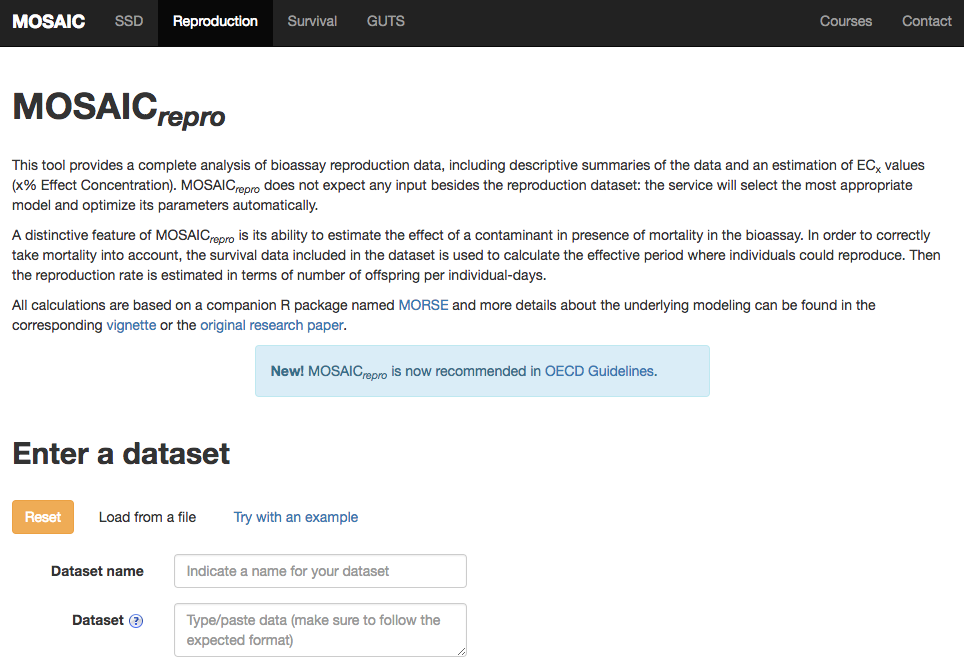## User data

You can upload your own data (click on Choose file) by taking care about the format specification of your file. MOSAIC$$_{repro}$$ expects to receive data as a tabular text file. Each line of the table corresponds to a time point for a given replicate and a given concentration of the contaminant, and provides one survival measurement (ie, a number of survivors) as well as one reproduction measurement (ie, a number of offspring). The table must contain the five following columns:

• replicate: a number or a string that is unique for each replicate;
• conc: the concentration of the contaminant;
• time: the time point of the measurement;
• Nsurv: the number of survivors;
• Nrepro: the number of offspring.

Please note that the first line of the file must contain column headings.

Once your data uploaded, you can choose the Concentration unit (ie, the x-label) as well as the y-label of plots in later results.

## Example data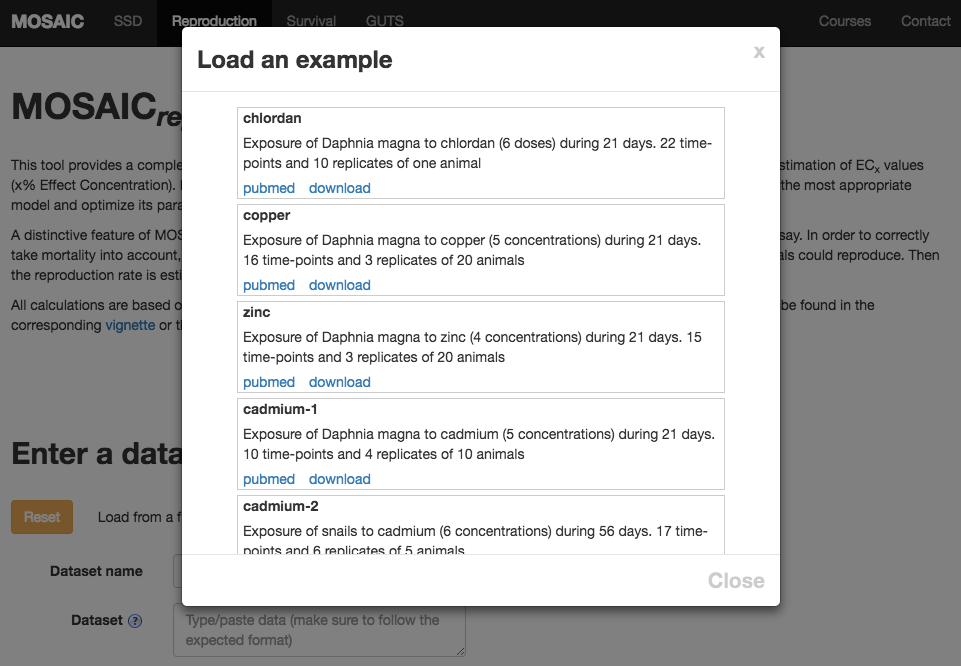In order to try MOSAIC$$_{repro}$$ with an example data set, choose the tab menu Try with an example. Choose cadmium-2 to get the same results as in this tutorial. Then, click Run.

# Step 2: Results and interpretation

After clicking on Run, you immediately see a summary of the experimental design corresponding to the data you uploaded.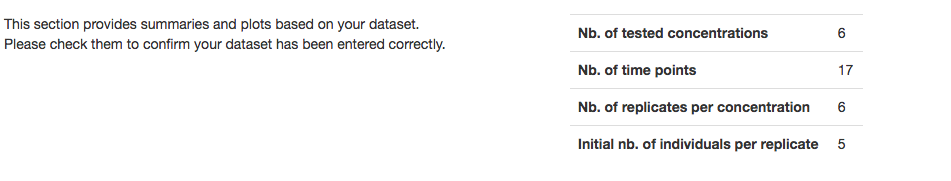You also get plots of survival and reproduction raw data:

• The number of survivors over time for each replicate and each concentration.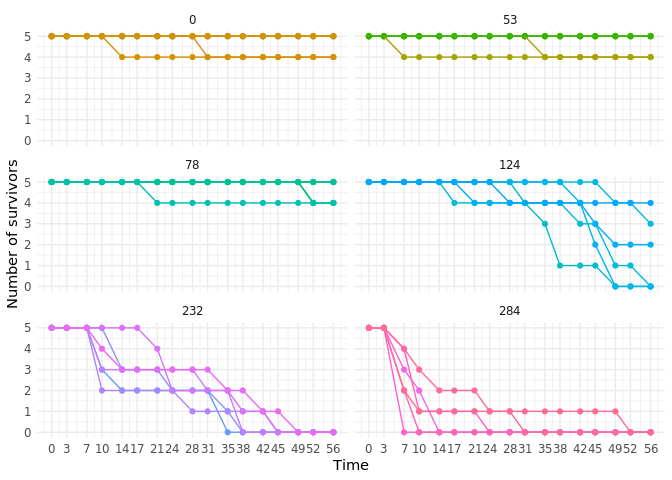• The observed fraction of surviving animals at final time at each tested contaminant concentration (black dots) together with the 95% binomial confidence intervals (black vertical segments).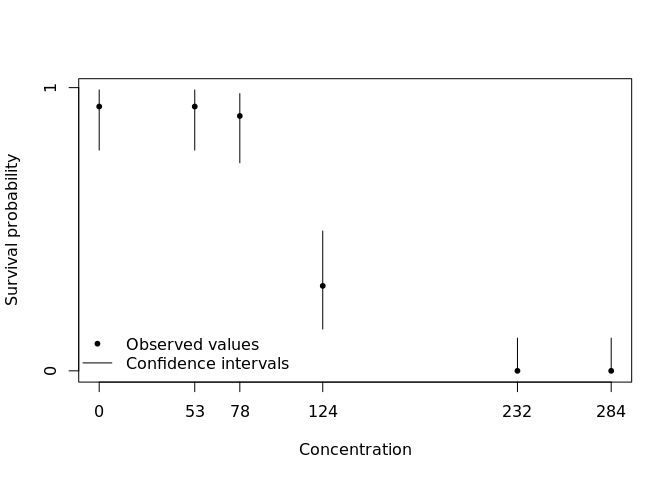• The number of offspring over time for each replicate and each concentration.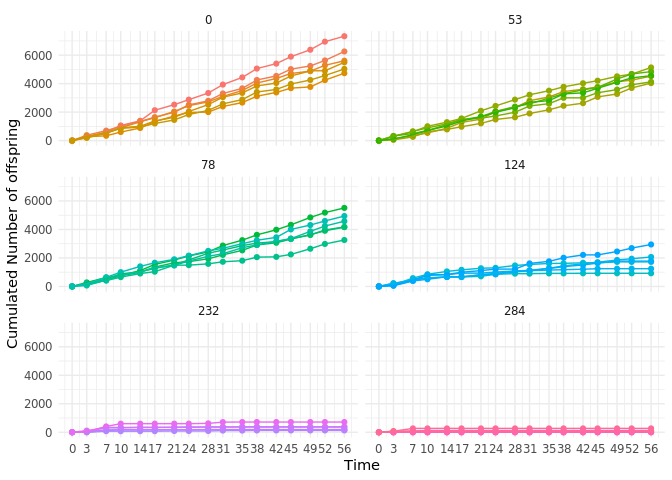• The observed reproduction rate at final time at each tested contaminant concentration (black dots) together with the 95% Poisson confidence intervals (black vertical segments).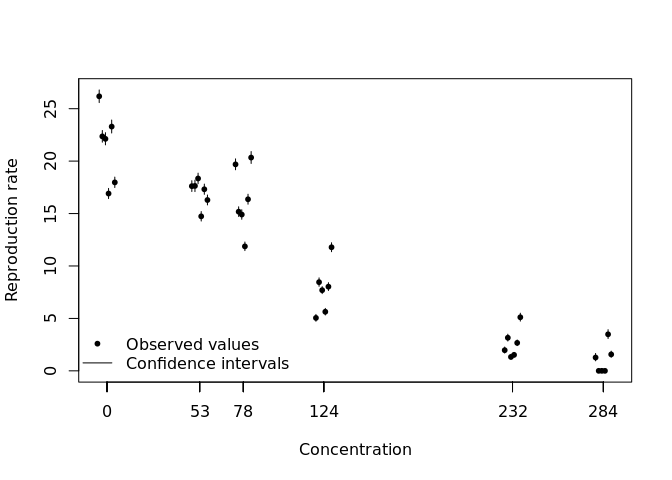Then you get the fitting results:

• The fitting plot which shows the mean number of offspring per individual-day as a function of the contaminant concentration (orange plain line) as well as the 95% credible band around this mean (light gray zone delimited by orange dotted lines). Black dots correspond to observed data while black vertical segments stand for the between-replicate variability (95% Poisson confidence intervals).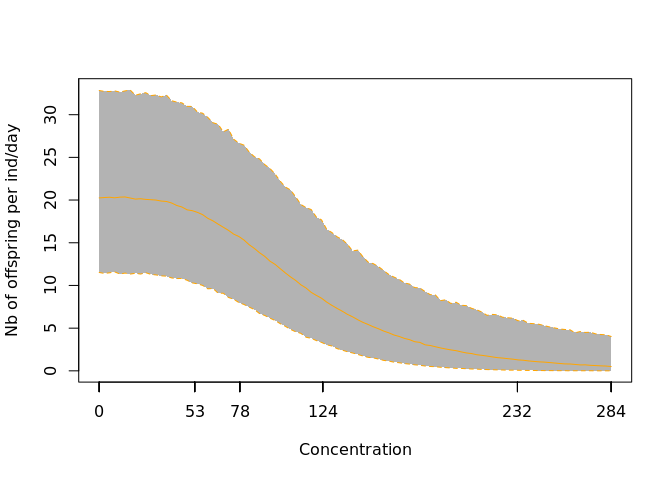• Model parameter estimates: we report the median (as the estimated value) and the 2.5% and 97.5% quantiles of the posterior (as a measure of uncertainty, a.k.a. 95% credible intervals).
            50%      2.5%     97.5%
b     3.366e+00 2.606e+00 4.321e+00
d     2.071e+01 1.837e+01 2.325e+01
e     1.131e+02 9.570e+01 1.329e+02
omega 1.256e+00 7.173e-01 2.421e+00

Take care about the value of parameter $$e$$ corresponding to the $$EC_{50}$$ estimate. If bounds are higher than the highest tested concentration, the value has to be considered with cautious.

• $$EC_x$$ estimates: we report the median (as the estimated value) and the 2.5% and 97.5% quantiles (as a measure of uncertainty, a.k.a. 95% credible intervals) of several $$EC_x$$ estimates, $$x = 1,...,80$$.
     median  Q2.5 Q97.5
EC1    28.8  17.4  44.0
EC5    47.1  32.5  65.0
EC10   58.8  42.9  77.6
EC15   67.4  51.0  86.7
EC20   74.8  58.0  94.2
EC25   81.5  64.5 101.0
EC30   87.8  70.6 108.0
EC40  100.0  82.9 120.0
EC50  113.0  95.7 133.0
EC60  128.0 110.0 148.0
EC70  146.0 128.0 167.0
EC80  171.0 151.0 196.0

As already stated before, the $$EC_{50}$$ estimate equals parameter $$e$$ estimate.

• The posterior predictive check (PPC): we plot the observed data against the corresponding predicted value from the fitted model as a 95% credible interval. If the fit is correct, we expect to see 95% of the observed values fall inside the credible intervals.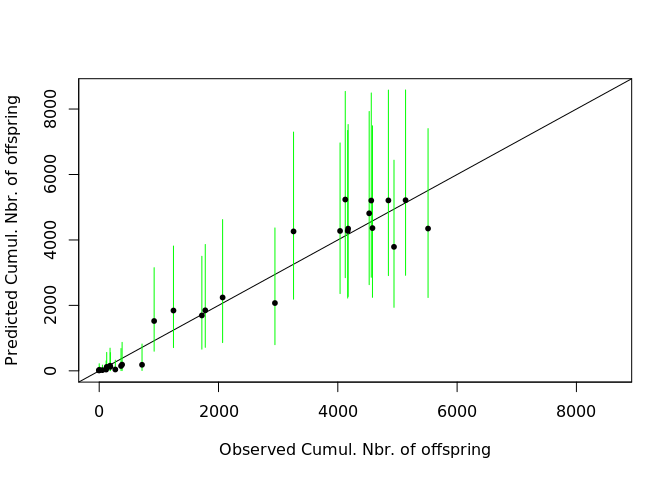Each black dot corresponds to one observation from the data set. The corresponding observed value can be read on the $$x$$-axis, while the $$y$$-axis reports the value predicted by the fitted model, as well as its 95% credible interval. The interval is colored in green if it contains the observed value, in red otherwise.

• R script: a gateway to perform further calculations directly within the R software .
library(morse)
summary(fit, quiet = TRUE)$Qpost signif(fit$estim.ECx, digits = 3)
ppc(fit, style = "generic")